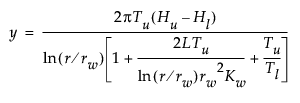Design of Experiments Guide > Space-Filling Designs > Example of a Sphere-Packing Design
Publication date: 11/10/2021

# Example of a Sphere-Packing Design

Worley (1987) presented a model of the flow of water through a borehole that is drilled from the ground surface through two aquifers. The response variable y is the flow rate through the borehole in m3/year and is determined by the following equation:There are eight factors in this model:

rw = radius of borehole, 0.05 to 0.15 m

r = radius of influence, 100 to 50,000 m

Tu = transmissivity of upper aquifer, 63,070 to 115,600 m2/year

Hu = potentiometric head of upper aquifer, 990 to 1100 m

Tl = transmissivity of lower aquifer, 63.1 to 116 m2/year

Hl = potentiometric head of lower aquifer, 700 to 820 m

L = length of borehole, 1120 to 1680 m

Kw = hydraulic conductivity of borehole, 9855 to 12,045 m/year

You can use a sphere-packing design to obtain a set of conditions for which to calculate the response y. Then you can build a model to estimate the true model over the range of inputs used in your design. Evaluation of your estimated model can help you understand the impact of each of the eight factors on the response.